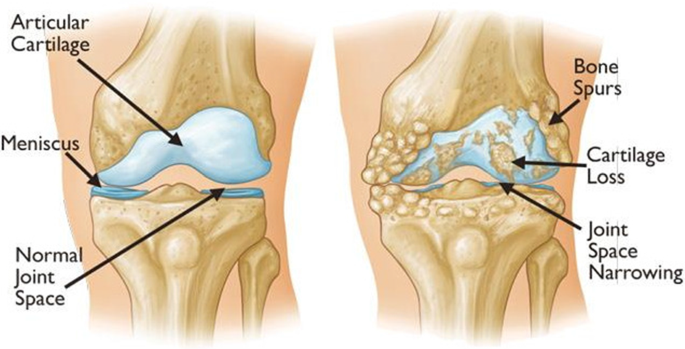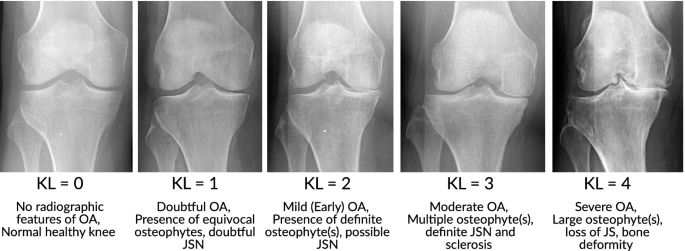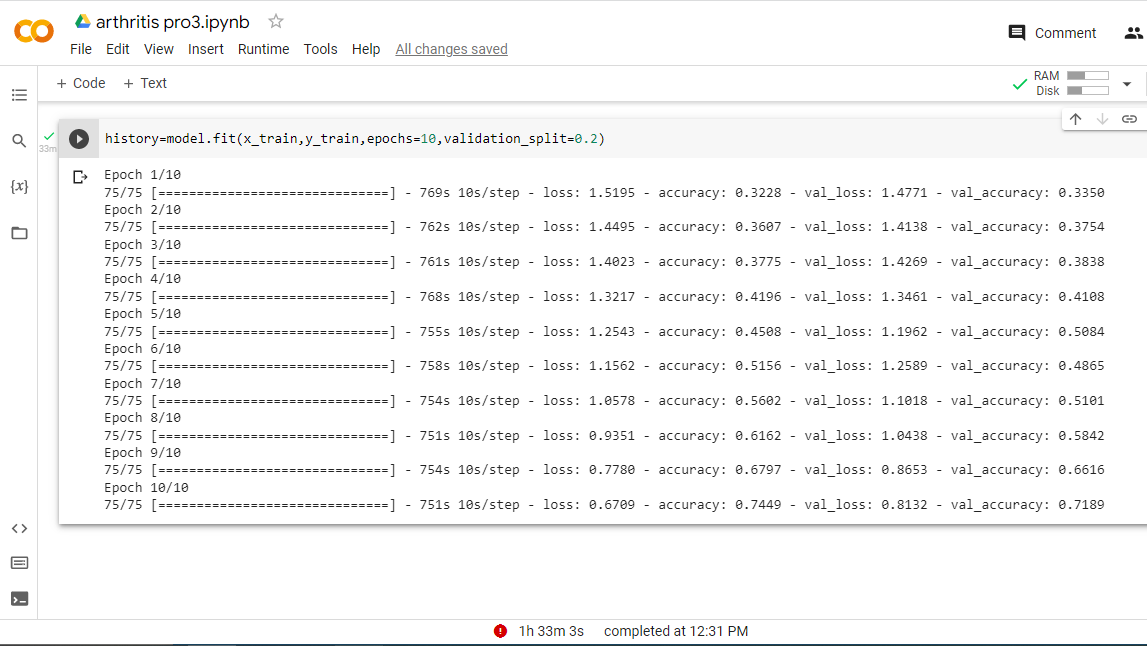# OSTEOARTHRITIS DETECTION IN KNEE USING CNN

Arthritis is one of the disorder that causes swelling, stiffness, tenderness and disability etc. It may be happen at one or more joints of the people. Arthritis is more common in older people and typically worsens with age. It is gradually increases in globally day by day. More than 350 million people have arthritis globally, this accounts for almost 4.43% of the human population. Although as of now there is no known cure for arthritis, the benefits of early detection can’t be understated.Sample of Normal and Osteoarthritis KneeThe KL grading system to assess the severity of knee OA.
• A convolution tool that separates and identifies the various features of the image for analysis in a process called as Feature Extraction.
• A fully connected layer that utilizes the output from the convolution process and predicts the class of the image based on the features extracted in previous stages.
1. Convolutional Layer :- This layer is the first layer that is used to extract the various features from the input images.
2. Pooling Layer :- In most cases, a Convolutional Layer is followed by a Pooling Layer. The primary aim of this layer is to decrease the size of the convolved feature map to reduce the computational costs.
3. Fully Connected Layer :- The Fully Connected (FC) layer consists of the weights and biases along with the neurons and is used to connect the neurons between two different layers. These layers are usually placed before the output layer and form the last few layers of a CNN Architecture.
4. Dropout :- Usually, when all the features are connected to the FC layer, it can cause overfitting in the training dataset. To overcome this problem, a dropout layer is utilised wherein a few neurons are dropped from the neural network during training process resulting in reduced size of the model.
5. Activation Functions :- Finally, one of the most important parameters of the CNN model is the activation function. They are used to learn and approximate any kind of continuous and complex relationship between variables of the network. In simple words, it decides which information of the model should fire in the forward direction and which ones should not at the end of the network.
`data_path=’/content/drive/MyDrive/Knee-project/Knee-Dataset/’categories=os.listdir(data_path)labels=[i for i in range(len(categories))]label_dict=dict(zip(categories,labels)) #empty dictionaryprint(label_dict)print(categories)print(labels)`
• data_path − This is the directory, which needs to be explored.
• os.listdir() method in python is used to get the list of all files and directories in the specified directory.
• for i in range() iterates through numbers. for element in my_list iterates through whatever is in the list (they could be numbers, too) for i in range(len(a_list)) iterates through numbers (which can be used for index access of a_list ).
• When using `LabelEncoder` to encode categorical variables into numerics,
• how does one keep a dictionary in which the transformation is tracked?
• i.e. a dictionary in which I can see which values became :- `{'A':1,'B':2,'C':3}`
`gray = cv2.cvtColor(img, cv2.COLOR_BGR2GRAY)resized=cv2.resize(gray,(img_size,img_size))`

# Defining Our Neural Network

`model=Sequential()model.add(Conv2D(128,(3,3),input_shape=data.shape[1:]))model.add(Activation(‘relu’))model.add(MaxPooling2D(pool_size=(2,2)))#The first CNN layer followed by Relu and MaxPooling layersmodel.add(Conv2D(64,(3,3)))model.add(Activation('relu'))model.add(MaxPooling2D(pool_size=(2,2)))#The second convolution layer followed by Relu and MaxPooling layersmodel.add(Conv2D(32,(3,3)))model.add(Activation('relu'))model.add(MaxPooling2D(pool_size=(2,2)))#The thrid convolution layer followed by Relu and MaxPooling layersmodel.add(Flatten())#Flatten layer to stack the output convolutions from 3rd convolution layermodel.add(Dropout(0.2))model.add(Dense(128,activation='relu'))#Dense layer of 128 neuronsmodel.add(Dropout(0.1))model.add(Dense(64,activation='relu'))#Dense layer of 64 neuronsmodel.add(Dense(5,activation='softmax'))#The Final layer with two outputs for two categories`
1. The first hidden layer `Conv2D` is a convolutional layer that has 128 feature maps, each with a size of 3 x 3 and we are using a rectified linear activation function - `relu`
2. We then add another convolutional layer with 64 feature maps, each with a size of 3 x 3 and we are using a rectified linear activation function — `relu`
3. We add a third convolutional layer with 32 feature maps, each with a size of 3 x 3 and we are using a rectified linear activation function — `relu`
4. We then add a pooling layer `MaxPooling2D1` that is configured with a pool size of 2 x 2. Max pooling operation for 2D spatial data. Down samples the input along its spatial dimensions (height and width) by taking the maximum value over an input window (of size defined by pool_size ) for each channel of the input.
5. We then convert the 2-dimensional matrix into a vector using `Flatten` - this allows our output to be processed by fully connected layers. Flattening is used to convert all the resultant 2-Dimensional arrays from pooled feature maps into a single long continuous linear vector. The flattened matrix is fed as input to the fully connected layer to classify the image.
6. We then apply a regularization layer using `Dropout` that is set to randomly exclude 20% of the neurons in the layer - this is used to reduce overfitting.
7. Next, we add a fully connected layer that has 128 neurons and a ReLU activation function.
8. We will then add another regularization layer to reduce overfitting, this time we’re randomly excluding 10% of the neurons.
9. Next, we add a fully connected layer that has 64 neurons and a ReLU activation function.
10. We finish the neural network with an output layer that has 5 neurons — the same as the number of classes in our classification problem and a softmax activation function. This will output a prediction of the probability that a digit belongs to each class.

# Compiling the Model

Before training the model we need to compile it and it define the loss function, optimizers, and metrics for prediction.

`model.compile(loss=’categorical_crossentropy’,optimizer=’adam’,metrics=[‘accuracy’])`
1. classification
2. regression
2. Stochastic Gradient Descent
4. Mini-Batch Gradient Descent
• Mean Absolute Error (MAE),
• Mean Squared Error (MSE),
• Root Mean Squared Error (RMSE),
• R² (R-Squared).
• Accuracy
• Confusion Matrix (not a metric but fundamental to others)
• Precision and Recall
• F1-score
• AU-ROC

# Add Dense layers on top

To complete the model, we will feed the last output tensor from the convolutional base (of shape (60, 60, 32)) into one or more Dense layers to perform classification. Dense layers take vectors as input (which are 1D), while the current output is a 3D tensor. First, we will flatten (or unroll) the 3D output to 1D, then add one or more Dense layers on top. Arthritis classification has 5 output classes, so you use a final Dense layer with 5 outputs.

1. Arrange the data
`from sklearn.model_selection import train_test_split`
`x_train,x_test,y_train,y_test=train_test_split(data,new_label,test_size=0.1)`
`history=model.fit(x_train,y_train,epochs=10,validation_split=0.2)`Accuracy in training and testing Phase
`mkdir app.pycd app.pypython3 -m venv venvsource venv/bin/activate`
`pip install flask`
`mkdir templates`
`set FLASK_APP=app.py      # this it the code  for windows.run flask`

# Prediction of Knee Osteoarthritis Results:-

When someone select and submit the Knee X-ray images, the webpage should display the predicted value of osteoarthritis i.e Normal, Doubtful, Mild, Moderate, Severe. For this, we require the model file (model.h1) we created before in the same project folder.

`<!doctype html><html><body> <h1> {{ prediction of Knee Osteoarthritis }}</h1></body></html>`

--

--